Simple-layer potential

An expression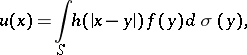(1)

whereis a closed Lyapunov surface (of class, cf. Lyapunov surfaces and curves) in the Euclidean space,, separatinginto an interior domainand an exterior domain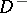;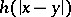is a fundamental solution of the Laplace operator: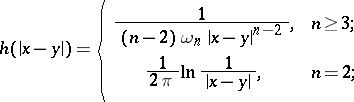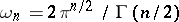is the area of the unit sphere in;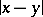is the distance between two pointsand; andis the area element on.

If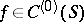, thenis everywhere defined on. A simple-layer potential is a particular case of a Newton potential, generated by masses distributed onwith surface density, and with the following properties.

In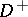anda simple-layer potentialhas derivatives of all orders, which can be calculated by differentiation under the integral sign, and satisfies the Laplace equation,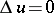, i.e. it is a harmonic function. Forit is a function regular at infinity,. A simple-layer potential is continuous throughout, and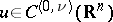for any,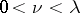. When passing through the surface, the derivative along the outward normal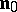toat a point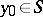undergoes a discontinuity. The limit values of the normal derivative fromandexist, are everywhere continuous on, and can be expressed, respectively, by the formula:(2)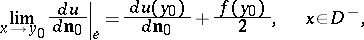where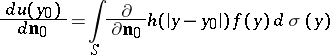(3)

is the so-called direct value of the normal derivative of a simple-layer potential at a point. Moreover,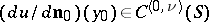for all,. If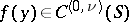, then the partial derivatives ofcan be continuously extended to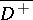andas functions of the classes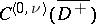and, respectively. In this case one also has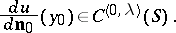These properties can be generalized in various directions. E.g., if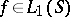, then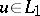inside and on, formulas (2) hold almost everywhere on, and the integral in (3) is summable on. One has also studied properties of simple-layer potentials understood as integrals with respect to arbitrary Radon measuresconcentrated on: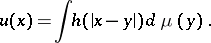Here, also,is a harmonic function outside, and formulas (2) hold almost everywhere onwith respect to the Lebesgue measure, where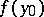is replaced by the derivative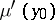of the measure. In definition (1) one can replace the fundamental solution of the Laplace equation by an arbitrary Lewy function of a general second-order elliptic operator with variable coefficients of class, replacing the normal derivativeby the derivative along the co-normal. The properties listed remain true in this case (cf. , , ).

Simple-layer potentials are used in solving boundary value problems for elliptic equations. The solution of a second boundary value problem with prescribed normal derivative is represented as a simple-layer potential with unknown density; the use of (2) and (3) leads to a Fredholm integral equation of the second kind onfor(cf. ).

In solving boundary value problems for parabolic equations one uses simple-layer heat potentials, of the form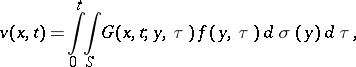where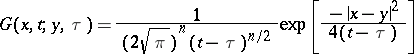is the fundamental solution of the heat equation in the-dimensional space, and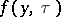is the density. The functionand its generalization to arbitrary second-order parabolic equations have properties analogous to those indicated for(cf. , , ).